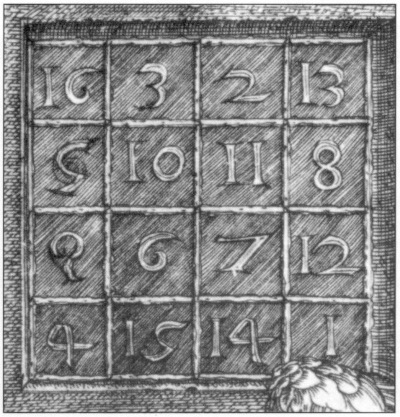# More MagicAlbrecht Dürer’s 1514 engraving Melencolia I includes this famous magic square: The magic sum of 34 can be reached by adding the numbers in any row, column, diagonal, or quadrant; the four center squares; the four corner squares; the four numbers clockwise from the corners; or the four counterclockwise.

In Power Play (1997), University of Toronto mathematician Ed Barbeau points out that there’s even more magic when we consider squares and cubes. Take the numbers in the first two and the last two rows:

16 + 3 + 2 + 13 + 5 + 10 + 11 + 8 = 9 + 6 + 7 + 12 + 4 + 15 + 14 + 1

162 + 32 + 22 + 132 + 52 + 102 + 112 + 82 = 92 + 62 + 72 + 122 + 42 + 152 + 142 + 12

Or alternate columns:

16 + 5 + 9 + 4 + 2 + 11 + 7 + 14 = 3 + 10 + 6 + 15 + 13 + 8 + 12 + 1

162 + 52 + 92 + 42 + 22 + 112 + 72 + 142 = 32 + 102 + 62 + 152 + 132 + 82 + 122 + 12

Most amazingly, if you compare the numbers on and off the diagonals, this works with both squares and cubes:

16 + 10 + 7 + 1 + 13 + 11 + 6 + 4 = 2 + 3 + 5 + 8 + 9 + 12 + 14 + 15

162 + 102 + 72 + 12 + 132 + 112 + 62 + 42 = 22 + 32 + 52 + 82 + 92 + 122 + 142 + 152

163 + 103 + 73 + 13 + 133 + 113 + 63 + 43 = 23 + 33 + 53 + 83 + 93 + 123 + 143 + 153